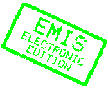MATHEMATICA BOHEMICA, Vol. 130, No. 2, pp. 167-176 (2005)

# A note on surfaces with radially symmetric nonpositive Gaussian curvature

## Joseph Shomberg

Joseph Shomberg, Department of Mathematical Sciences, University of Wisconsin-Milwaukee, P.O. Box 413, Milwaukee, WI 53201, USA, e-mail: shomberg@uwm.eduAbstract: It is easily seen that the graphs of harmonic conjugate functions (the real and imaginary parts of a holomorphic function) have the same nonpositive Gaussian curvature. The converse to this statement is not as simple. Given two graphs with the same nonpositive Gaussian curvature, when can we conclude that the functions generating their graphs are harmonic? In this paper, we show that given a graph with radially symmetric nonpositive Gaussian curvature in a certain form, there are (up to) four families of harmonic functions whose graphs have this curvature. Moreover, the graphs obtained from these functions are not isometric in general.

Keywords: Gaussian curvature, holomorphic function

Classification (MSC2000): 53A05, 35C05

Full text of the article:

[Previous Article] [Next Article] [Contents of this Number] [Journals Homepage]
© 2005–2010 FIZ Karlsruhe / Zentralblatt MATH for the EMIS Electronic Edition